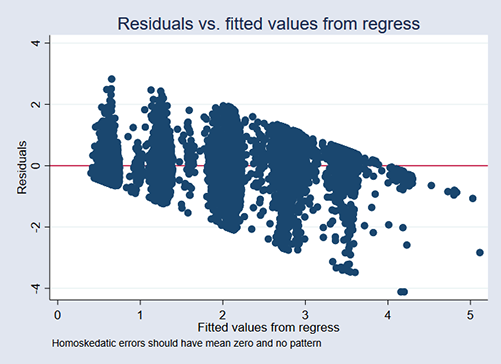»  Home »  Products »  Features »  Heteroskedastic linear regression

## Heteroskedastic linear regression

### Highlights

• Linear regression with multiplicative heteroskedastic errors
• Flexible exponential function for the variance
• Maximum likelihood estimator
• Two-step GLS estimator
• Support for Bayesian estimation
• Robust, cluster–robust, and bootstrap standard errors
• Complex survey designs support

hetregress fits linear regressions in which the variance is an exponential function of covariates that you specify. It allows you to model the heteroskedasticity. When we fit models using ordinary least squares (regress), we assume that the variance of the residuals is constant. If it is not constant, regress reports biased standard errors, leading to incorrect inferences. hetregress lets you deal with the heterogeneity.

Modeling the variance as an exponential function also produces more efficient parameter estimates if the variance model is correctly specified.

hetregress implements two estimators for the variance: a maximum likelihood (ML) estimator and a two-step GLS estimator. The ML estimates are more efficient than those obtained by the GLS estimator if the mean and variance function are correctly specified and the errors are normally distributed. The two-step GLS estimates are more robust if the variance function is incorrect or the errors are nonnormal.

### Let's see it work

We model students' high school performance (grade point average or GPA) as a function of

• their attendance rate (attend)
• whether they are freshmen, sophomores, juniors, or seniors
• their participation in sports (sports)
• their participation in after school activities
• whether they take advanced placement courses (ap)
• whether they are boys (boy)
• their parent's maximum level of educational attainment (pedu)

We could fit the model by typing

. regress  gpa  attend i.(grade sports extra ap boy pedu)


After fitting the model, we found evidence of heteroskedasticity using the existing postestimation command estat hettest, which did not surprise us. We suspected that the variance might increase with the student's grade level if nothing else. As students age, they become different. We had suspicions about the effects of other variables as well.So we refit the model using hetregress:

. hetregress gpa attend i.(grade sports extra ap boy pedu),

Fitting full model:

Iteration 0:   log likelihood = -8244.2526
Iteration 1:   log likelihood = -8146.4604
Iteration 2:   log likelihood = -8143.9845
Iteration 3:   log likelihood = -8143.9825
Iteration 4:   log likelihood = -8143.9825

Heteroskedastic linear regression               Number of obs     =     10,000
ML estimation
Wald chi2(10)     =   49185.25
Log likelihood = -8143.983                      Prob > chi2       =     0.0000

gpa   Coefficient  Std. err.      z    P>|z|     [95% conf. interval]

gpa
attend     .6315888   .0471474    13.40   0.000     .5391816    .7239961

sophomore     -.0043576    .010086    -0.43   0.666    -.0241257    .0154105
junior     -.0161349     .01465    -1.10   0.271    -.0448484    .0125787
senior     -.0124978   .0201447    -0.62   0.535    -.0519806    .0269851

sports
yes      .7129917   .0147291    48.41   0.000     .6841232    .7418601

extra
yes      .7025737   .0152534    46.06   0.000     .6726776    .7324697

ap
yes      .3651225   .0283152    12.89   0.000     .3096258    .4206192

boy
boy     -.7186189    .008559   -83.96   0.000    -.7353942   -.7018435

pedu
college      1.558124   .0092734   168.02   0.000     1.539948    1.576299
graduate      2.468524   .0191345   129.01   0.000     2.431021    2.506027

_cons     .7233421   .0432877    16.71   0.000     .6384998    .8081844

lnsigma2
sophomore      .8428276   .0402258    20.95   0.000     .7639864    .9216688
junior      1.765285   .0403254    43.78   0.000     1.686249    1.844322
senior      2.539946   .0396568    64.05   0.000      2.46222    2.617672

pedu
college      .7894325   .0305812    25.81   0.000     .7294945    .8493705
graduate      .9831641   .0512158    19.20   0.000     .8827831    1.083545

ap
yes      .1425211   .0898203     1.59   0.113    -.0335234    .3185656

extra
yes     -.0339061   .0530107    -0.64   0.522    -.1378052     .069993

ap#extra
yes#yes      .7684617   .3065945     2.51   0.012     .1675476    1.369376

attend     .0946848   .1562184     0.61   0.544    -.2114977    .4008672
_cons    -3.057355   .1452952   -21.04   0.000    -3.342129   -2.772582

LR test of lnsigma2=0: chi2(9) = 4778.76                  Prob > chi2 = 0.0000

The coefficients under the heading gpa compose our main model for the mean of gpa.

The coefficients under the heading lnsigma2 are the coefficients of the exponential model for the variance.

The likelihood-ratio test reported at the bottom of the table tells us that our model of the variance fits the data better than a model where the variance is constant.